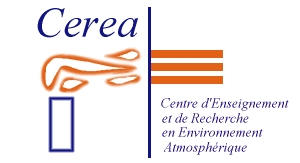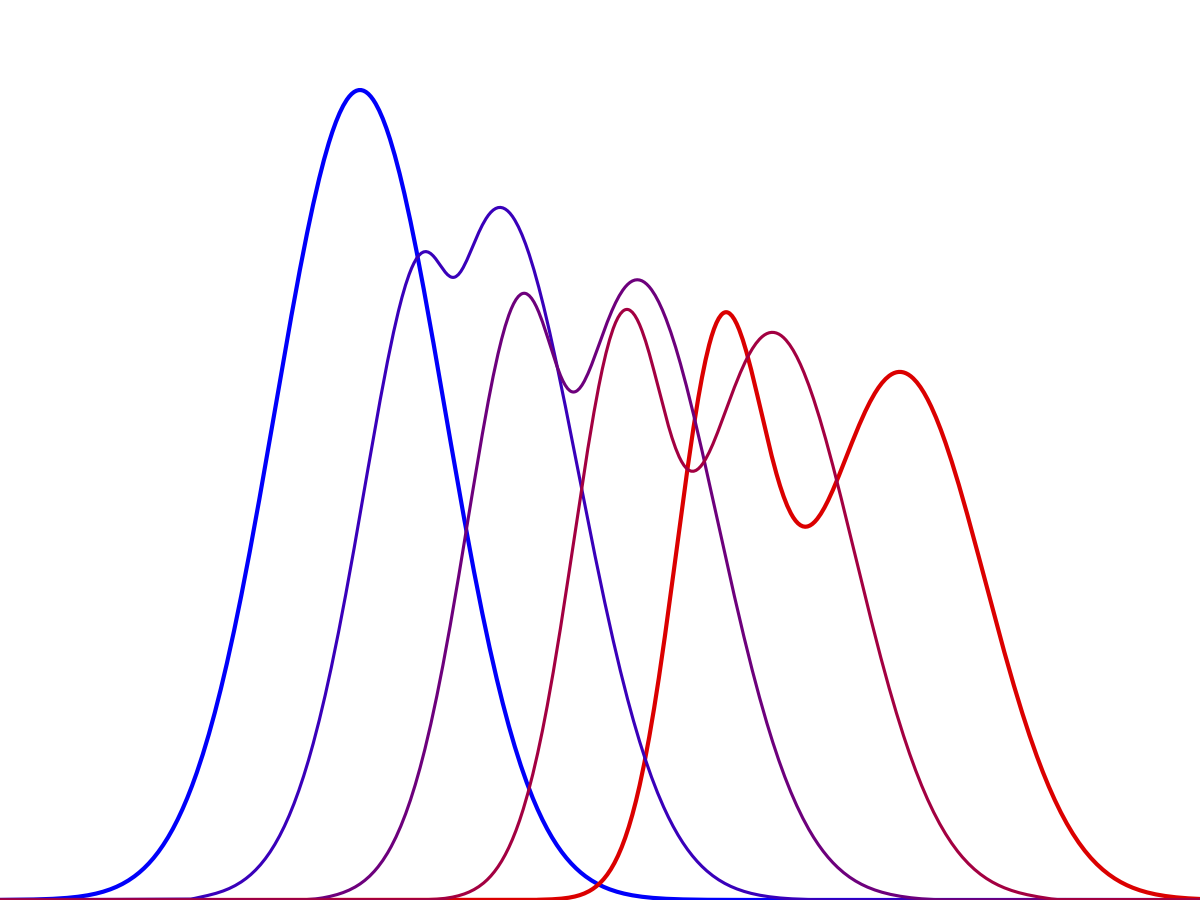Optimal transport problem solver The optimal transport theory is the study of optimal transportation and allocation between measures. The optimal transport problem was first introduced by Monge (1781) and formalized by Kantorovitch (1942), leading to the so called Monge-Kantorovitch transportation problem. The goal is to look for a transport map transforming a probability density function into another while minimizing the cost of transport.

• Brief description of the problem

Let $$\mathbb{E}$$ be a Euclidean space of dimension $$d$$ and let $$f_0$$ and $$f_1$$ be two probability density functions over $$\mathbb{E}$$.

We look for a couple density-velocity $$\left(f,v\right)$$ defined over $$\mathbb{E}\times[0,1]$$ satisfying a continuity constraint $\forall \left(\mathbf{x},t\right)\in\left(\mathbb{E}\times[0,1]\right), \quad \frac{\partial f}{\partial t}\left(\mathbf{x},t\right) + \mathrm{div}\left(f\mathbf{v}\right)\left(\mathbf{x},t\right) = 0,$ a boundary condition $\forall \mathbf{x}\in\mathbb{E}, \quad f\left(\mathbf{x},t=0\right) = f_0(\mathbf{x}) \quad \text{and} \quad f\left(\mathbf{x},t=1\right) = f_1(\mathbf{x}),$ and minimizing the cost of transport $J\left(f,\mathbf{v}\right) = \int_{\mathbb{E}\times[0,1]} f\left(\mathbf{x},t\right) \cdot \left\| \mathbf{v}\left(\mathbf{x},t\right) \right\|^2 \rm{d}\mathbf{x}\rm{d}t.$

The square-root of the minimum of this cost function is defined as the Wasserstein distance between $$f_0$$ and $$f_1$$. The argument $$f^*$$ of the minimum defines a non-trivial interpolation between $$f_0$$ and $$f_1$$.

For more details on this problem see (Benamou and Brenier, 2000; Farchi et al., 2016).

The optimal transport problem is a minimization problem under constraint. Following Papadakis et al. (2014), we proposed iterative solvers that rely on the use of proximal operators.

In the python code we implemented the Douglas-Rachford and the Primal-Dual algorithms in order to compute the optimal density and velocity field as defined above given two tables representing discretized versions of the probability density functions $$f_0$$ and $$f_1$$. The python code can handle the one-dimensional and two-dimensional cases ($$d=1$$ or $$2$$); it is object-oriented; it heavily relies on the standard numpy and scipy modules (e.g. linalg, fftpack, tensordot...); and it is not parallelized.

For more details about the code see (Farchi et al., 2016).

• Application to atmospheric dispersion

Farchi et al. (2016) discusses the relevance of using the Wasserstein distance as an indicator to discriminate between fields of pollutant in the context of the Fukushima-Daiichi nuclear power plant accident. In this paper, the authors used this solver to solve the optimal transport problem and to compute the Wasserstein distance.